﻿ Law of Cosines

# LAW OF COSINES

• PRACTICE (online exercises and printable worksheets)

To solve a triangle means to find all the angles and all the side lengths.

The Law of Sines is a valuable tool in solving triangles,
but the Law of Sines requires knowing an angle and its opposite side.

Therefore, we need a different tool to begin solving a SSS (side-side-side) or SAS (side-angle-side) configuration.

The Law of Cosines (which can be viewed as a generalization of the Pythagorean Theorem) comes to the rescue!

 In this section, capital letters are used to denote angles/vertices. Corresponding lowercase letters are used to denote lengths of opposite sides. Thus: angle $\,D\,$ is opposite side $\,d\,$ angle $\,E\,$ is opposite side $\,e\,$ angle $\,F\,$ is opposite side $\,f\,$ To significantly cut down on words, I say things like: ‘angle $\,D\,$ is opposite side $\,d\,$’ instead of the more correct (but wordier): ‘angle $\,D\,$ is opposite the side of length $\,d\,$’ See more issues about triangle notation here.## Motivation for the Law of Cosines

 Consider the triangle at right. Since $\,D\,$ is a right angle, the well-known Pythagorean Theorem tells us: $$d^2 = e^2 + f^2$$ Such a beautiful, simple, relationship between the lengths of the sides: $$\overbrace{d^2\strut }^{\text{the square of the longest side}} \overbrace{=\strut }^{\text{is}} \overbrace{e^2 + f^2\strut }^{\text{the sum of the squares of the shorter sides}}$$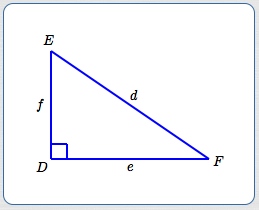But, what if $\,D\,$ isn't a right angle? Let's investigate. Keep $\,e\,$ and $\,f\,$ the same, but change angle $\,D\,$: Make $\,D\,$ bigger, so it's now an obtuse angle. To compensate, its opposite side needs to get longer: $$d_{\text{new}} > d$$ Make $\,D\,$ smaller, so it's now an acute angle. To compensate, its opposite side needs to get shorter: $$d_{\text{new}} < d$$ The Law of Cosines introduces an ‘adjustment’ to the Pythagorean Theorem to account for the change in the length of $\,d\,$!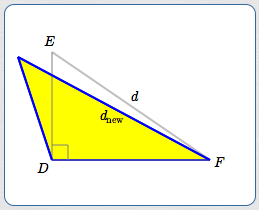If $\,D\,$ becomes an obtuse angle, its opposite side gets longer: $$d_{\text{new}} > d$$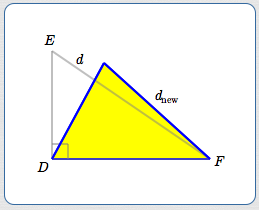If $\,D\,$ becomes an acute angle, its opposite side gets shorter: $$d_{\text{new}} < d$$

THEOREM the Law of Cosines
 Consider an arbitrary triangle (see right), where: angle $\,D\,$ is opposite a side of length $\,d\,$ angle $\,E\,$ is opposite a side of length $\,e\,$ angle $\,F\,$ is opposite a side of length $\,f\,$ Then: $$d^2 = e^2 + f^2 - 2ef\cos D$$## Notes on the Law of Cosines:

• Three Applications of the Law of Cosines in Every Triangle:
Since there are three sides in every triangle,
there are three applications of the Law of Cosines in every triangle.
Here are the other two versions: $$\begin{gather} e^2 = d^2 + f^2 - 2df\cos E\cr\cr f^2 = d^2 + e^2 - 2de\cos F \end{gather}$$
• Verbalizing the Law of Cosines:
In any triangle, the square of a side is the sum of the squares of the other two sides,
minus twice the product of the other two sides times the cosine of the included angle.
Quite a mouthful!
Here are the words, ‘attached’ to the formula: $$\overbrace{d^2\strut}^{\text{the square of a side}} \overbrace{=\strut}^{\text{is}} \underbrace{e^2 + f^2\strut}_{\text{the sum of the squares of the other two sides}} \overbrace{-\strut}^{\text{minus}} \underbrace{2ef\strut}_{\text{twice the product of the other two sides}} \overbrace{\cdot\strut}^{\text{times}} \underbrace{\cos D\strut}_{\text{the cosine of the included angle}}$$
Here is the ‘adjustment’ in the Law of Cosines: $$d^2 = e^2 + f^2 \ \overbrace{- 2ef\cos D}^{\text{the ‘adjustment’}}$$ When the adjustment is positive, then $\,d^2\,$ is bigger than it would be, if it were opposite a $\,90^\circ\,$ angle.
A bigger value of $\,d^2\,$ means a bigger value of $\,d\,$. $$d^2 \quad=\quad e^2 + f^2 \ \ \overbrace{- 2ef\cos D}^{\text{positive}} \quad>\quad e^2 + f^2$$ Similarly, when the adjustment is negative, then $\,d^2\,$ is smaller than it would be, if it were opposite a $\,90^\circ\,$ angle.
A smaller value of $\,d^2\,$ means a smaller value of $\,d\,$. $$d^2 \quad=\quad e^2 + f^2 \ \ \overbrace{- 2ef\cos D}^{\text{negative}} \quad < \quad e^2 + f^2$$
• When $\,D = 90^\circ$, the Adjustment is Zero:

When $\,D = 90^\circ\,$ (that is, in a right triangle), we have $\,\cos D = \cos 90^\circ = 0\,$.
In this case, the adjustment is zero, and the Law of Cosines reduces to the Pythagorean Theorem.
• When $\,D\,$ is Obtuse, the Adjustment is Positive:
 For angles $\,D\,$ strictly between $\,90^\circ\,$ and $\,180^\circ\,$, we have $\,\cos D < 0\,$. Since $\,e\,$ and $\,f\,$ (side lengths) are strictly positive, this means that $$\text{the adjustment} \quad=\quad \overbrace{-2\strut}^{(-)} \ \overbrace{ef\strut}^{(+)} \ \overbrace{\cos D\strut}^{(-)} \quad > \quad 0$$ Since the adjustment is positive, $\,d\,$ is longer than it would be, if it were opposite a $\,90^\circ\,$ angle. Take another look at the image from the motivation (at right)!• When $\,D\,$ is Acute, the Adjustment is Negative:
 For angles $\,D\,$ strictly between $\,0^\circ\,$ and $\,90^\circ\,$, we have $\,\cos D > 0\,$. Since $\,e\,$ and $\,f\,$ (side lengths) are strictly positive, this means that $$\text{the adjustment} \quad=\quad \overbrace{-2\strut}^{(-)} \ \overbrace{ef\strut}^{(+)} \ \overbrace{\cos D\strut}^{(+)} \quad < \quad 0$$ Since the adjustment is negative, $\,d\,$ is shorter than it would be, it if were opposite a $\,90^\circ\,$ angle. Take another look at the image from the motivation (at right)!## Proof of the Law of Cosines

The proof of the Law of Cosines is a smidgeon harder than the proof of the Law of Sines,
but it's not too bad for such a powerful result.

 Here's a re-naming of rectangular coordinates that is needed in the proof: Take any point $\,P(x,y)\,$ in the plane. Recall that $\,P(x,y)\,$ is a convenient notation for a point $\,P\,$ with coordinates $\,(x,y)\,$. Let $\,r\,$ denote the distance from the origin to $\,P\,$. Let $\,\theta\,$ be any angle (measured from the positive $x$-axis) with terminal side passing through $\,P\,$. By similar triangles (see the sketch at right): $$\begin{gather} x = r\cos\theta\cr y = r\sin\theta \end{gather}$$ So, $\,P(x,y) = P(r\cos\theta,r\sin\theta)\,$. (This is a useful re-naming in many mathematical situations!)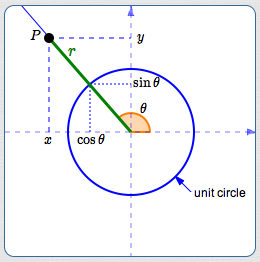Now, here's the derivation of the Law of Cosines:
 Position the triangle in a coordinate plane with one vertex at the origin and one side on the $x$-axis. Figure out the coordinates of the other two (non-origin) vertices. (Use the result above to find the coordinates for $\,F\,$.) Use the distance formula to find the distance between the two (non-origin) vertices. So that we don't have to deal with square roots, square both sides of the distance formula: $$\text{distance between (x_1,y_1) and (x_2,y_2)} = \sqrt{(x_2-x_1)^2 + (y_2-y_1)^2}$$ to get: $${(\text{distance})}^2 = (x_2-x_1)^2 + (y_2-y_1)^2$$ Note that the distance from $\,E(f,0)\,$ to $\,F(e\cos D,e\sin D)\,$ is $\,d\,$. Also recall the Pythagorean Identity, which states that $\,\cos^2 \theta + \sin^2\theta = 1\,$ for all angles $\,\theta\,$. Thus: \begin{alignat}{2} d^2 &= (e\cos D - f)^2 + (e\sin D - 0)^2 &&\text{(distance formula)}\cr\cr &= e^2\cos^2 D - 2ef\cos D + f^2 + e^2\sin^2 D &\qquad&\text{(FOIL)}\cr\cr &= e^2(\cos^2 D + \sin^2 D) + f^2 - 2ef\cos D&&\text{(factor, re-arrange terms)}\cr\cr &= e^2(1) + f^2 - 2ef\cos D&&\text{(Pythagorean Identity)}\cr\cr &= e^2 + f^2 - 2ef\cos D&&\text{Voila!!} \end{alignat} QED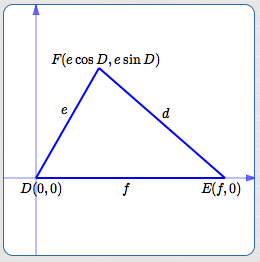## Example: Using the Law of Cosines in a SAS Configuration

Consider a triangle with side lengths $\,3\,$ and $\,5\,$, and an included $\,57^\circ\,$ angle.
Prove that this information uniquely defines a triangle, and then solve the triangle.

If using a calculator, store the full accuracy of computed sides/angles.
Then, use these (most accurate) stored values in intermediate calculations.

Summarize the values of sides and angles in a table.
In the table, round values to one decimal place.

In the solution below, the default accuracy provided by WolframAlpha is reported and used for intermediate calculations.

## Solution

By SAS, the triangle is unique.
The notation at right is used in the solution.
• By the Law of Cosines: $$\begin{gather} d^2 = 3^2 + 5^2 - 2\cdot 3\cdot 5\cdot\cos 57^\circ\cr\cr d^2 = 34 - 30\cos 57^\circ\cr\cr d \approx 4.202 \end{gather}$$ Note: Computation of $\,d\,$ was done by typing in

sqrt(34 - 30cos(57 deg))

at WolframAlpha.
• Now that we have an angle and its opposite side,
we can use the Law of Sines. But we have to be careful!

If you're not careful, then you can proceed merrily along, seemingly doing everything right, and end up with a ‘triangle’ that doesn't actually exist. For example, you might notice that the longest side isn't opposite the biggest angle. Or—if you take the time to check the three Law of Sines ratios—they won't all be the same. A math emergency! It can be really hard to locate what has gone wrong in the universe.

The well-hidden problem that can occur has to do with the arcsine. When you have a statement like ‘$\,\sin E = 0.5988\,$’ and use the arcsine to find $\,E\,$ (look below—there's a star next to it), this step is fraught with peril! There are TWO angles between $\,0^\circ\,$ and $\,180^\circ\,$ (both of which could potentially be angles in a triangle) for which $\,\sin E = 0.5988\,$. The arcsine only gives you one of them—the acute one. Fine and dandy, if that's the one you want. If you're unlucky, you're on your way to a math emergency.

I'll illustrate such a conundrum in a future section. But, the problem can be avoided, and you need to start developing good habits now.
• In any triangle, when comparing angle/opposite side pairs, the shorter side will be opposite the smaller angle.
• Given any two angles in any triangle, one of them must be acute. (Why? Otherwise, the angle sum would be greater than $\,180^\circ\,$.)
• When you use the Law of Sines in a situation where you want an acute angle, it will give you the one you want.
• When you have a choice, always use the Law of Sines with the shortest side possible .
Okay, now we can get back to work! $$\begin{gather} \frac{\sin 57^\circ}{4.202} = \frac{\sin E}{3} \qquad (\text{Use the shorter side!})\cr\cr \sin E = \frac{3\sin 57^\circ}{4.202} \approx 0.5988\cr\cr E = \arcsin(0.5988) \approx 36.78^\circ \qquad \bigstar \end{gather}$$
• $\,F = 180^\circ - 57^\circ - 36.78^\circ = 86.22^\circ$
• Summarize values (with requested rounding) in a table:  ANGLES SIDES $D = 57^\circ$ $d = 4.2$ $E = 36.8^\circ$ $e = 3$ $F = 86.2^\circ$ $f = 5$
• Use both a rough sketch and the Law of Sines to do a ‘sanity check’.
According to the sketch, the values are reasonable.
Are the following ratios all approximately the same? $$\begin{gather} \frac{\sin 57^\circ}{4.2} \approx 0.1997 \cr\cr \frac{\sin 36.8^\circ}{3} \approx 0.1997 \cr\cr \frac{\sin 86.2^\circ}{5} \approx 0.1996 \cr\cr \end{gather}$$ Okay! (Slight differences may occur due to approximations.)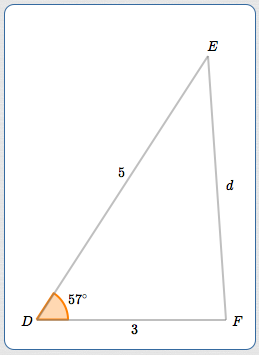If you (like me) want everything to be ‘just perfect’ in your mathematical world,
then you may have been bothered by the usage of ‘$\,=\,$’ and ‘$\,\approx\,$’ in the previous example.